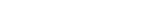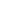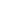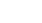How Cheenta works to ensure student success?
Explore the Back-Story

# Number of zeroes after factorial |TOMATO Objective 154The numberends exactly with

(A)zeroes;

(B)zeroes;

(C)zeroes;

(D)zeroes;
Discussion:

To find the number of zeroes at the end of n! we just need to figure out the number of 5's occurring in prime factorization of it.  Why? Because there are much more 2's present than there are 5's (every second number is even hence contributing at least one 2 to the prime factorization). Every 0 at the end of n! is basically one 10 made of one 2 and one 5.

Every fifth number contributes at least one 5.  This allows for= 200 fives.

Again every 25th number contributes an extra 5. This allows for= 40 extra fives.

Every 125th number contributes another extra 5. This allows for= 8 more extra fives.

Finally every 625th number contribute one more extra 5. This allows for= 1 more extra fives.

Hence there are total 249 fives in the prime factorization of 1000!. Hence it ends with 249 zeroes.

The numberends exactly with

(A)zeroes;

(B)zeroes;

(C)zeroes;

(D)zeroes;
Discussion:

To find the number of zeroes at the end of n! we just need to figure out the number of 5's occurring in prime factorization of it.  Why? Because there are much more 2's present than there are 5's (every second number is even hence contributing at least one 2 to the prime factorization). Every 0 at the end of n! is basically one 10 made of one 2 and one 5.

Every fifth number contributes at least one 5.  This allows for= 200 fives.

Again every 25th number contributes an extra 5. This allows for= 40 extra fives.

Every 125th number contributes another extra 5. This allows for= 8 more extra fives.

Finally every 625th number contribute one more extra 5. This allows for= 1 more extra fives.

Hence there are total 249 fives in the prime factorization of 1000!. Hence it ends with 249 zeroes.

This site uses Akismet to reduce spam. Learn how your comment data is processed.

### 2 comments on “Number of zeroes after factorial |TOMATO Objective 154”

1.Ansuman Mandal says:

Please correct that anubhav air that first term should be  ,but it is given .....

2.Anubhab says:

Thank you Ansuman...It has been edited..!

### Knowledge Partner